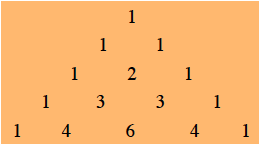### Home > PC3 > Chapter 12 > Lesson 12.2.1 > Problem12-100

12-100.

Use Pascal’s triangle to expand $(a + bc)^4$. Hint: Expand $(x + y)^4$ and then substitute the $a$ for $x$ and $bc$ for $y$.

Use Pascal's triangle to get the coefficients quickly.Substitute $(bc)$ for $y$ with the ( ).
You will make fewer careless errors!